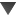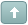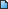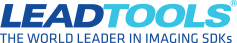Error

#Loading 12 bit grayscale images

#1 Posted : Tuesday, October 31, 2017 1:38:08 PM(UTC)
Hi,

I have a question about how to handle 12 bit grayscale images.

We use a proprietary image format so I handle all of the image loading.
The raw data we get is a 16 bit image with the high bit set to 11 and the image
data ranging from 0 to 4095. How would I go about creating a RasterImage
from this?

This is how I currently load our 16 bit images.

RaasterImage img = RasterImage.CreateGrayscale(m_width, m_height, m_bpp, m_dpi); // m_bpp is 16

I then load the data manually using SetRow()

for (int count = 0; count < m_height; count++)
{
img.SetRow(count, m_imageData, count * m_width * (m_bpp / 8),
m_imageSize / m_height);
}

How does LeadTools store 12 bit data internally? I was assuming I still needed 2 bytes per pixel value
so I would have a 16 bit image with 12 bit values but that setup has caused problems with some of the filters.

Thank you

Tony

 Try the latest version of LEADTOOLS for free for 60 days by downloading the evaluation: https://www.leadtools.com/downloadsWanna join the discussion? Login to your LEADTOOLS Support accountor Register a new forum account.#2 Posted : Friday, November 3, 2017 1:44:50 PM(UTC)
Hello Tony,

After a some searching, I was able to find this old forum post about 12-bit grayscale images: https://www.leadtools.com/support/forum/posts/t4697-12-bit-Grayscale-images. Below are some helper functions I made to convert between a row of shorts (though you can change the type to int) to the byte array the SetRow function is expecting.

Code:
``````
private byte[] CreatePair(short p1, short p2)
{
// p1 = 0xABC
// p2 = 0xDEF
// res = [ BC, FA, DE ]
return new byte[] { (byte)(p1 & 0xFF), (byte)(((p2 & 0xF) << 4) | ((p1 >> 8) & 0xF)), (byte)((p2 >> 4) & 0xFF) };
}
private byte[] CreateRow(short[] row)
{
List<byte> res = new List<byte>();

for (int i = 0; i < row.Length; i += 2)
{
byte[] pair;
if (i + 1 < row.Length)
pair = CreatePair(row[i], row[i + 1]);
else // Handle odd length row, use 0x000 for next pixel
pair = CreatePair(row[i], 0x000);

}

return res.ToArray();
}
``````

And here’s a sample of how to use it:

Code:
``````
private void Form1_Load(object sender, EventArgs e)
{
int bpp = 12;
int width = 5;
int height = 2;
int dpi = 1;

RasterImage image = RasterImage.CreateGrayscale(width, height, bpp, dpi);

// Gradient from black to white
short[] row = new short[] { 0x000, 0x444, 0x888, 0xCCC, 0xFFF };
byte[] row12 = CreateRow(row);
image.SetRow(0, row12, 0, row12.Length);
}
``````

Attached is the image this produces (see Screenshot_1.png).
File Attachment(s):CAS-62070-G5Z8T7.zip (2kb) downloaded 529 time(s).

Edited by moderator Tuesday, November 7, 2017 4:00:51 PM(UTC)  | Reason: Not specified

Anthony Northrup
Developer Support Engineer